# Regular polygon

Here you will learn about regular polygons, including what they are and how to classify them.

Students will first learn about regular polygons as part of geometry in 2nd grade and 3rd grade.

## What is a regular polygon?

A regular polygon is a closed, straight-sided figure that has equal side and angle measurements. If a polygon does not have all equal sides and interior angles, it is an irregular polygon.

For example,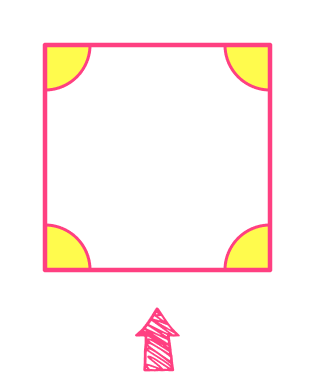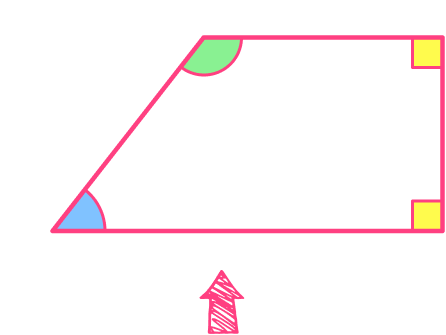All the sides are the same length All the sides are NOT the same length

All the angles are the same size All the angles are NOT the same size

The table below gives examples of regular polygons:

PolygonNumber of
sides/vertices
Image
(regular polygon)
Equilateral triangle 3
Regular pentagon 5
Regular hexagon 6
Regular octagon 8

### What are regular polygons?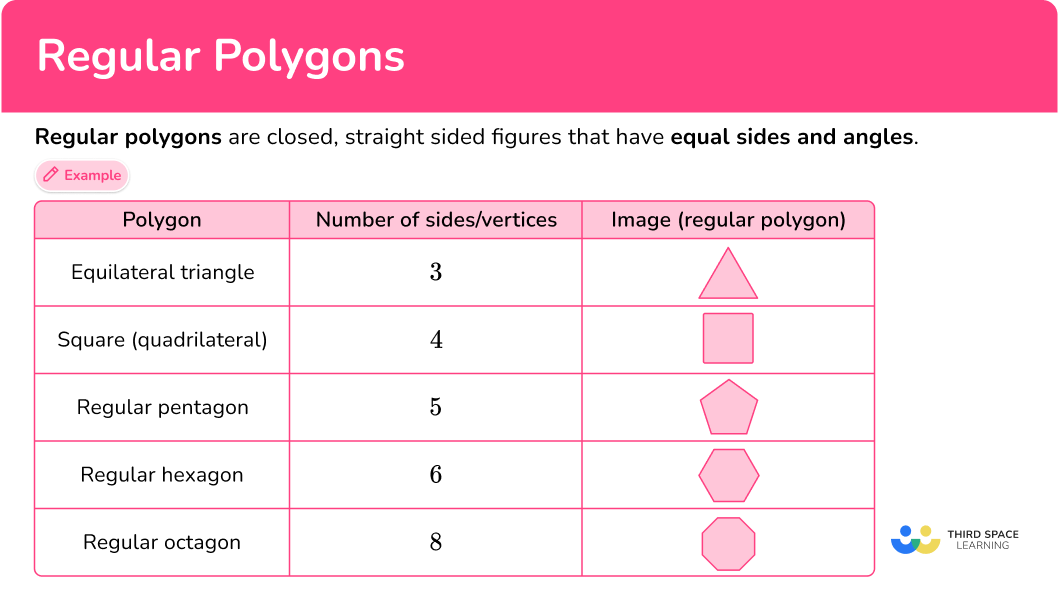## Common Core State Standards

How does this relate to 2nd grade math and 3rd grade math?

• Grade 2 – Geometry (2.G.A.1)
Recognize and draw shapes having specified attributes, such as a given number of angles or a given number of equal faces. Identify triangles, quadrilaterals, pentagons, hexagons, and cubes.

• Grade 3 – Geometry (3.G.A.1)
Understand that shapes in different categories (example, rhombuses, rectangles, and others) may share attributes (example, having four sides), and that the shared attributes can define a larger category (example, quadrilaterals).

Recognize rhombuses, rectangles, and squares as examples of quadrilaterals, and draw examples of quadrilaterals that do not belong to any of these subcategories.

## How to classify a regular polygon

In order to classify a regular polygon:

1. Check to see that the polygon has congruent (equal) angles and sides.
2. Count the number of sides of the polygon.
3. Name the polygon based on the number of sides.

## Regular polygon examples

### Example 1: classify the shape – by sides and regular or irregular

Name the shape.

1.  Check to see that the polygon has congruent (equal) angles and sides.

All the sides and angles are congruent, so the polygon is regular.

2Count the number of sides of the polygon.

There are 4 sides.

3Name the polygon based on the number of sides.

The polygon is a regular quadrilateral or more specifically a square.

### Example 2: classify the shape – by sides and regular or irregular

Name the shape.

All the sides and angles are congruent, so the polygon is regular.

There are 3 sides.

The polygon is a regular triangle or more specifically an equilateral triangle.

### Example 3: classify the shape – by sides and regular or irregular

Name the shape.

All the sides and angles are congruent, so the polygon is regular.

There are 6 sides.

The polygon is a regular hexagon.

### Example 4: classify the shape – by sides and regular or irregular

Name the shape.

All the sides and angles are congruent, so the polygon is regular.

There are 5 sides.

The polygon is a regular pentagon.

### Example 5: classify the shape – by sides and regular or irregular

Name the shape.

All the sides and angles are congruent, so the polygon is regular.

There are 8 sides.

The polygon is a regular octagon.

### Example 6: classify the shape – by sides and regular or irregular

Name the shape.

All the sides and angles are congruent, so the polygon is regular.

There are 4 sides.

The polygon is a regular quadrilateral or more specifically a square.

### Teaching tips for regular polygons

• Give students opportunities to physically create regular polygons, not just draw or identify them. A simple activity with straws of the same lengths and clay can be used. Identify the straws as line segments and the clay as vertices. Then students can use them to create their own regular polygons.

• Instead of emphasizing quizzes, routinely highlight regular polygons that are common in everyday life (at home or at school) and encourage students to notice them as well. Daily practice over time helps students remember the definitions and also provides them with a variety of real life examples for each regular polygon.

### Easy mistakes to make

• Thinking a rectangle is a regular polygon
Since the sides of a regular polygon must be equal, a rectangle is not a regular polygon unless it has 4 equal sides – which is more specifically classified as a square.
For example,

• Thinking regular polygons can’t have diagonal lines
While polygons must have straight sides, the sides don’t have to be vertical (go up and down). They can also be diagonal (on a slant), such as in a regular triangle or pentagon.
For example,

### Practice regular polygon questions

1. Which of the following is a regular polygon?Regular polygons are closed, straight sided figures that have equal sides and angles.The figure is closed and all sides and angles are congruent.

2. Which of the following is a regular polygon?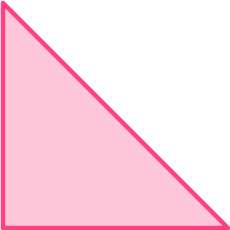Regular polygons are closed, straight sided figures that have equal sides and angles.The figure is closed and all sides and angles are congruent.

3. Which of the following is a regular hexagon?A regular hexagon is a 6 sided closed figure that has equal sides and angles.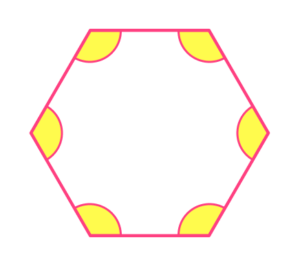4. Which of the following is a regular quadrilateral?A regular quadrilateral is a 4 sided closed figure that has equal sides and angles.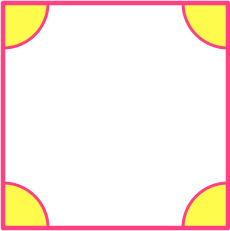5. Which object in the picture is a regular polygon?the clockthe windowthe mirrorthe pictureRegular polygons are closed, straight sided figures that have equal sides and angles.

The picture frame has equal sides and angles – it is a regular quadrilateral or a square.6. Which object in the picture is a regular polygon?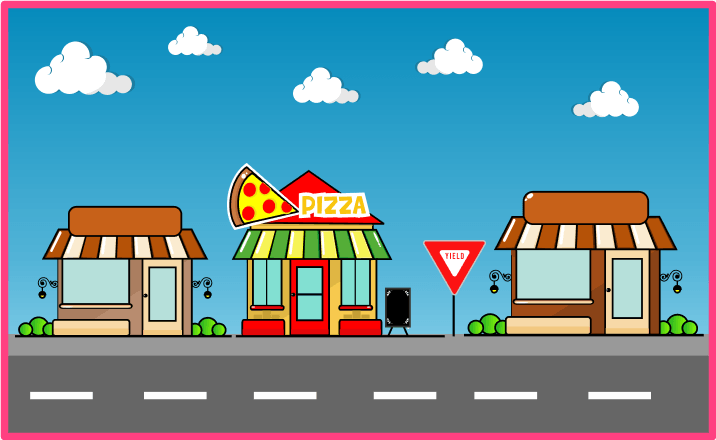the slice of pizzathe street signthe pizza store doorthe windows above the pizza storeRegular polygons are closed, straight sided figures that have equal sides and angles.

The street sign has equal sides and angles – it is a regular triangle or an acute, equilateral triangle.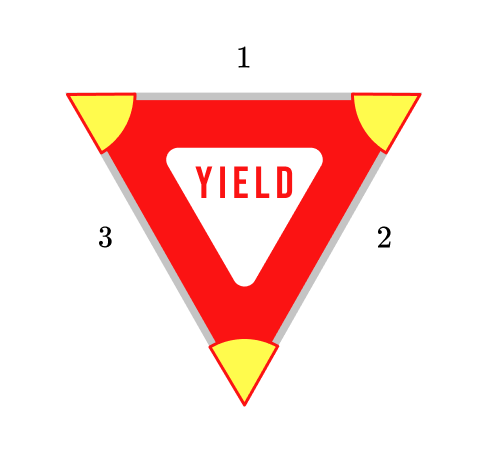## Regular polygon FAQs

What are the types of polygons?

There are two types – regular and irregular.

What is a regular polygon?

A closed figure made up of straight lines is a polygon. The properties of a regular polygon are: equal side lengths and equal angles (equiangular).

How do you find the perimeter of a regular polygon?

Since the sides are congruent, the perimeter is just one side length, n times (the number of sides in an n -sided polygon).

How do you find the area of a regular polygon?

There is a formula to find the area of all regular polygons, which comes from the area of triangles. However, it only works for regular polygons. To find the area of irregular polygons, you can break apart the polygon into common shapes, such as triangles or rectangles, to find the area.

What is the difference between a concave polygon and a convex polygon?

All the internal angles (interior angles) in a convex polygon are all less than 180 degrees. One or more of the internal angles in a concave polygon is greater than 180 degrees.

Are the exterior angles of a regular polygon also congruent?

Yes, for a regular polygon both the interior and exterior angles are all congruent.

What are other common polygons?

There are heptagons, which have seven sides, nonagons, which have nine sides and decagons, which have ten sides. There are also names for polygons with more than ten sides.

## Still stuck?

At Third Space Learning, we specialize in helping teachers and school leaders to provide personalized math support for more of their students through high-quality, online one-on-one math tutoring delivered by subject experts.

Each week, our tutors support thousands of students who are at risk of not meeting their grade-level expectations, and help accelerate their progress and boost their confidence.